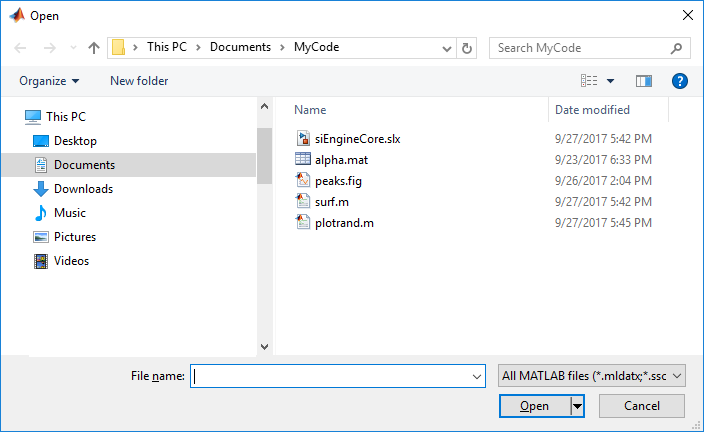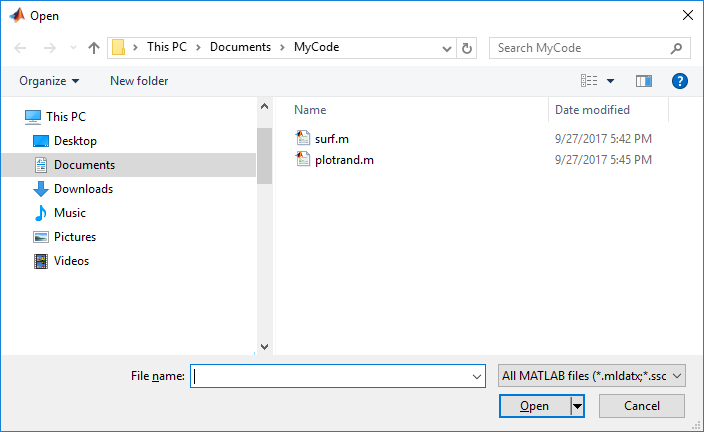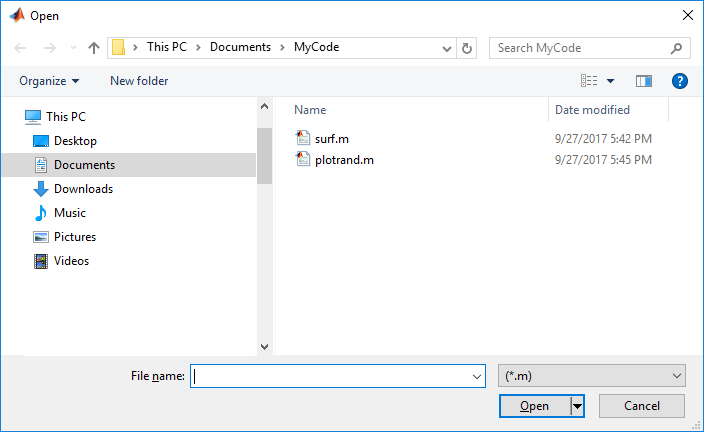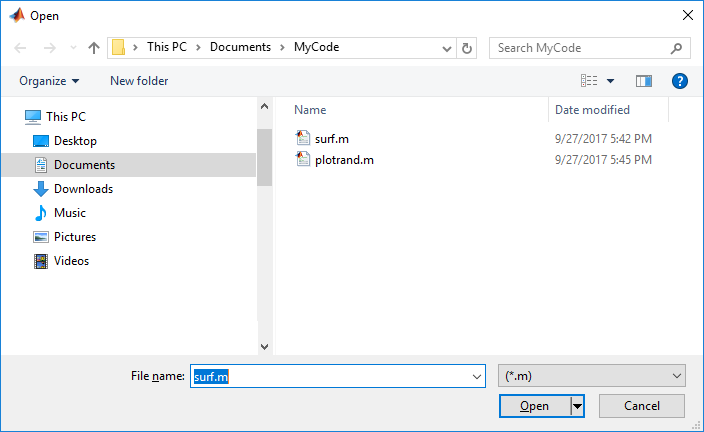# uiopen

## 语法

``uiopen``
``uiopen(type)``
``uiopen(file)``
``uiopen(file,tf)``

## 说明

````uiopen` 打开一个标题为“打开”的模态对话框。对话框中的文件筛选器设置为所有 MATLAB® 文件。如果用户在文件名字段中输入有效的文件名并点击打开，则 MATLAB 将在适用的应用程序中打开指定的文件。如果没有安装适用的应用程序，将在 MATLAB 编辑器中打开文件或者返回错误。 注意对话框的可视特征取决于运行代码的操作系统。例如，某些操作系统不在对话框中显示标题栏。 ```

``uiopen(type)` 根据指定的文件类型（与文件扩展名不同）设置文件筛选器。例如，如果类型为 `'figure'`，则 MATLAB 将筛选器设置为所有图形文件 `(*.fig)`。`

``uiopen(file)` 指定在对话框的文件名字段中显示的默认文件名。对话框中只显示与此默认文件名具有相同扩展名的文件。 如果指定通配符和文件扩展名（例如 `*.m`），则文件名字段不会显示任何文件名。对话框中只显示具有指定扩展名的文件。`type` 值出现在文件名字段右侧的筛选器字段中。`
``uiopen(file,tf)` 在 `tf` 的值为逻辑值 true (1) 时直接打开指定的文件而不显示“打开”对话框。在 `tf` 的值为逻辑值 false (0) 时，显示“打开”对话框。 `

## 示例

```uiopen ````type` 参数设置为 `'editor'`，以便只显示可在编辑器中打开的文件。对话框显示的列表中将排除 FIG 文件、MAT 文件、SLX 文件、MLAPP 文件和 MLAPPINSTALL 文件。

```uiopen('editor') ``````uiopen('*.m') ````file` 输入参数设置为某个文件的文件名。当对话框打开时，该文件将出现在文件名字段中。

```uiopen('surf.m') ```## 输入参数

`'matlab'`所有 MATLAB 文件。
`'load'`所有 MAT 文件 `(*.mat)`
`'figure'` 所有图窗文件 `(*.fig)`
`'simulink'`所有 Simulink® 模型文件（`*.mdl``*.slx`
`'editor'``.mat``.fig``.slx``.mlapp``.mlappinstall` 文件之外的所有 MATLAB 文件

## 提示

• 下表列出了文件扩展名和对应的应用程序（当用户选择具有该扩展名的文件并点击打开时，将在该应用程序中打开此文件）。如果计算机上未安装所列出的工具，而文件是一个文本文件，则将在 MATLAB 编辑器中打开该文件。如果未安装 Simulink，而用户选择了具有 `.mdl``.slx` 文件扩展名的文件，MATLAB 将返回错误。

文件扩展名加载文件的位置
`.m``.mlx` MATLAB 编辑器
`.fig`MATLAB 图窗窗口。
`.mat`MATLAB 工作区。
`.mlapp`

MATLAB App 设计工具。

`.mlappinstall` MATLAB App 安装程序
`.mdl``.slx`Simulink
• 要在 Microsoft® Windows® 中打开文件，请使用 `winopen` 函数。

## 备选方法

FIG 文件`openfig`
MAT 文件`load`